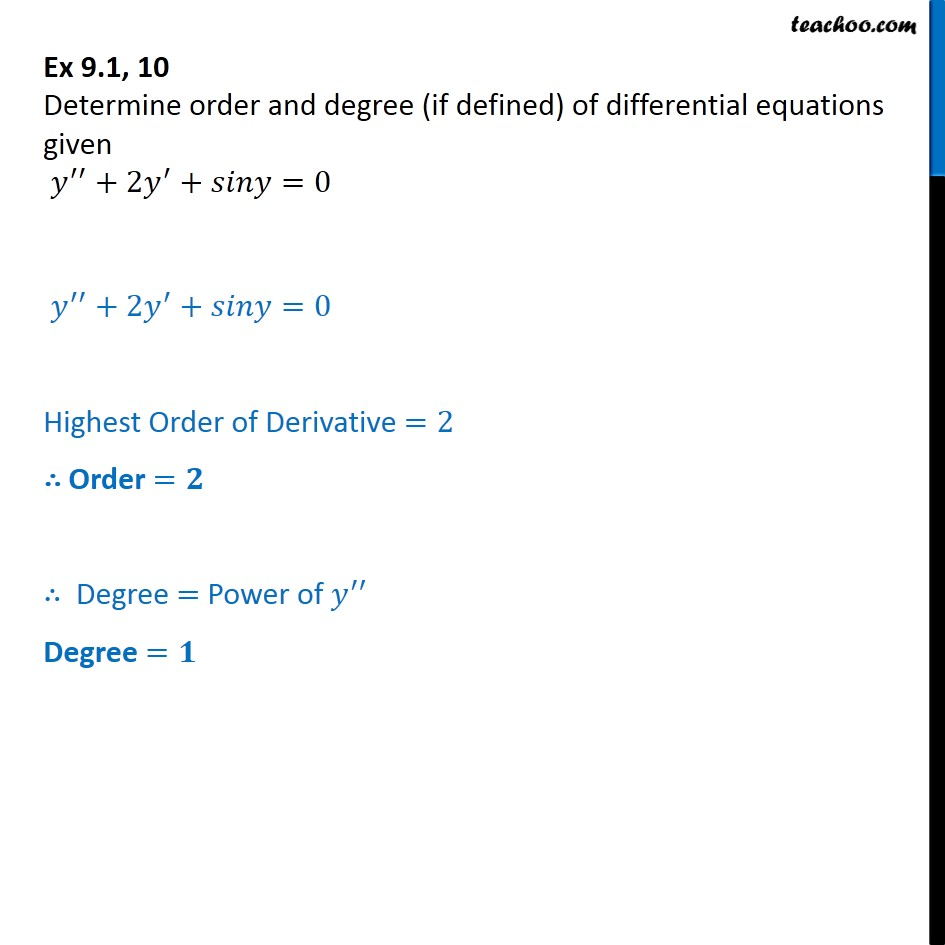Ex 9.1

Chapter 9 Class 12 Differential Equations
Serial order wiseLearn in your speed, with individual attention - Teachoo Maths 1-on-1 Class

### Transcript

Ex 9.1, 10 Determine order and degree (if defined) of differential equations given y + 2y + = 0 y + 2y + = 0 Highest Order of Derivative =2 Order = Degree = Power of y Degree =